# Competition Graphs of Semiorders Fred Roberts Rutgers University

• Slides: 62
Download presentationCompetition Graphs of Semiorders Fred Roberts, Rutgers University Joint work with Suh-Ryung Kim, Seoul National 1 UniversityHappy Birthday Jean-Claude !! 2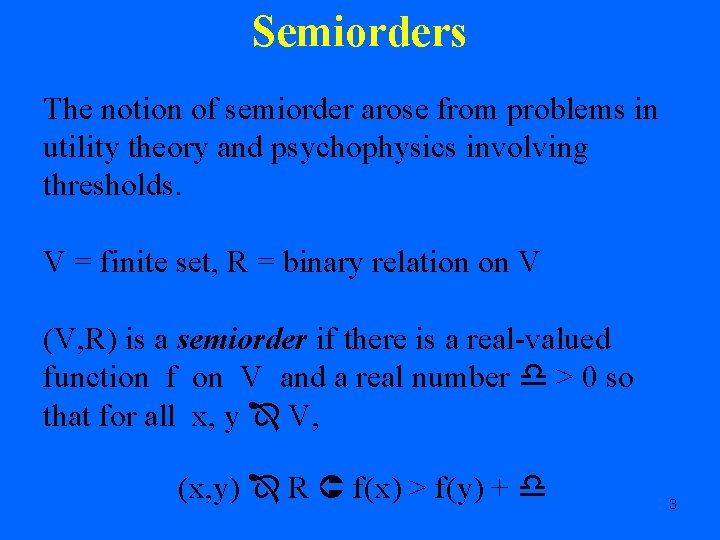Semiorders The notion of semiorder arose from problems in utility theory and psychophysics involving thresholds. V = finite set, R = binary relation on V (V, R) is a semiorder if there is a real-valued function f on V and a real number > 0 so that for all x, y V, (x, y) R f(x) > f(y) + 3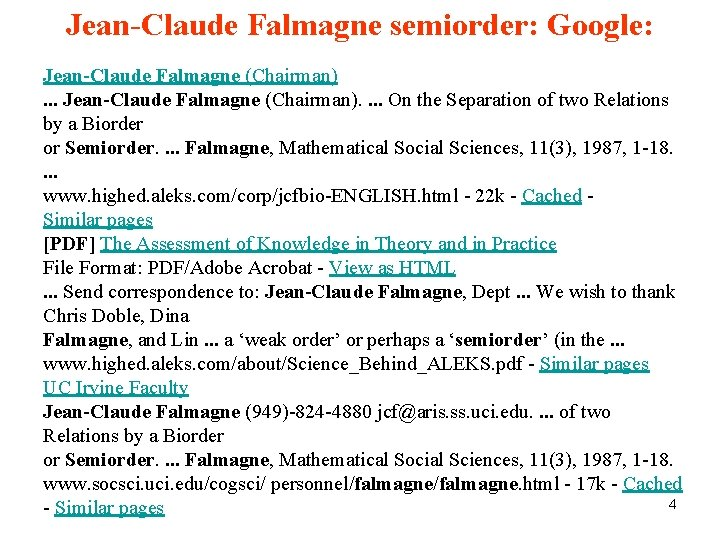Jean-Claude Falmagne semiorder: Google: Jean-Claude Falmagne (Chairman). . . . On the Separation of two Relations by a Biorder or Semiorder. . Falmagne, Mathematical Social Sciences, 11(3), 1987, 1 -18. . www. highed. aleks. com/corp/jcfbio-ENGLISH. html - 22 k - Cached - Similar pages [PDF] The Assessment of Knowledge in Theory and in Practice File Format: PDF/Adobe Acrobat - View as HTML. . . Send correspondence to: Jean-Claude Falmagne, Dept. . . We wish to thank Chris Doble, Dina Falmagne, and Lin. . . a ‘weak order’ or perhaps a ‘semiorder’ (in the. . . www. highed. aleks. com/about/Science_Behind_ALEKS. pdf - Similar pages UC Irvine Faculty Jean-Claude Falmagne (949)-824 -4880 [email protected] ss. uci. edu. . of two Relations by a Biorder or Semiorder. . Falmagne, Mathematical Social Sciences, 11(3), 1987, 1 -18. www. socsci. uci. edu/cogsci/ personnel/falmagne. html - 17 k - Cached 4 - Similar pagesJean-Claude Falmagne and Semiorders • 1987 paper with Doignon and Falmagne on separation of two orders by a biorder or semiorder. • Work on biorders goes way back – 1969 paper with Ducamp on composite measurement 5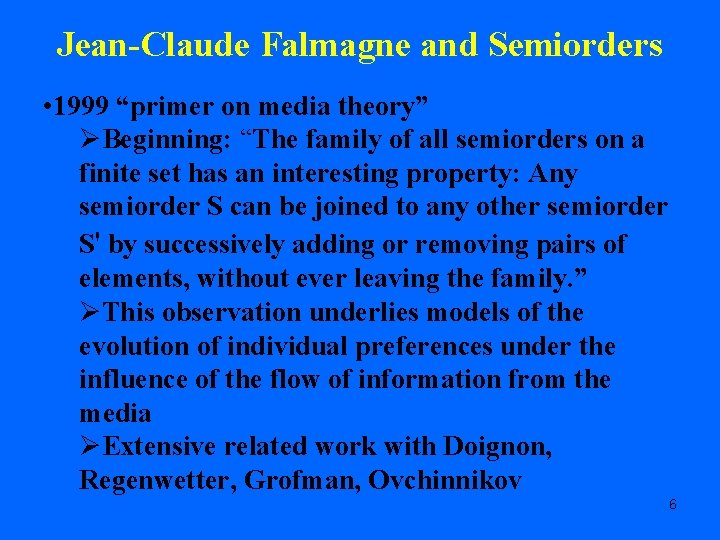Jean-Claude Falmagne and Semiorders • 1999 “primer on media theory” ØBeginning: “The family of all semiorders on a finite set has an interesting property: Any semiorder S can be joined to any other semiorder S' by successively adding or removing pairs of elements, without ever leaving the family. ” ØThis observation underlies models of the evolution of individual preferences under the influence of the flow of information from the media ØExtensive related work with Doignon, Regenwetter, Grofman, Ovchinnikov 6Jean-Claude Falmagne and Semiorders • A similar idea underlies the notion of well-graded families of relations (Doignon and Falmagne 1997) • 2001 paper with Doble, Doignon, and Fishburn on “almost connected orders” – based on one of the standard axioms for semiorders (no 3 -point chain is incomparable to a fourth point) • These are just a few examples. 7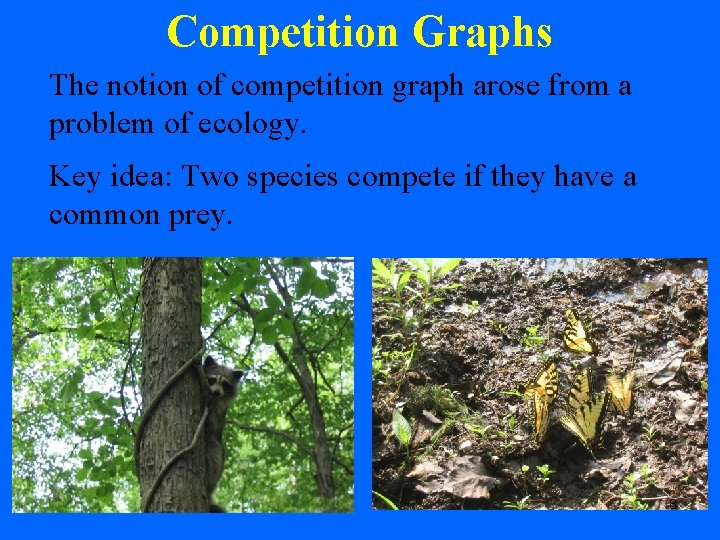Competition Graphs The notion of competition graph arose from a problem of ecology. Key idea: Two species compete if they have a common prey. 8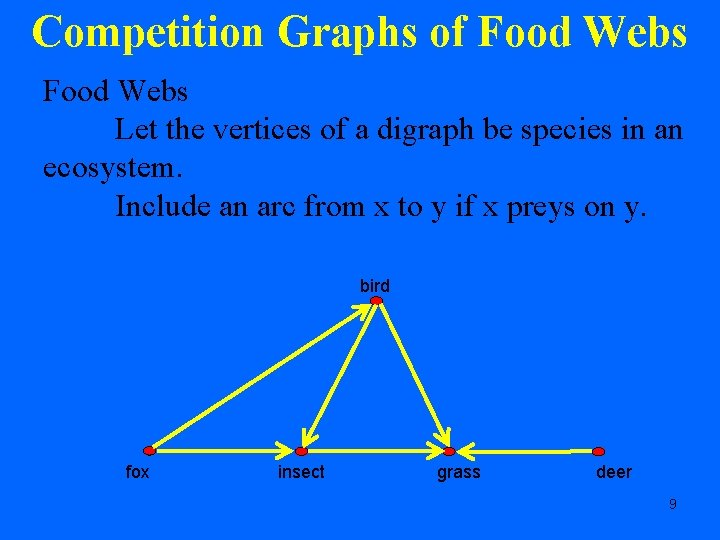Competition Graphs of Food Webs Let the vertices of a digraph be species in an ecosystem. Include an arc from x to y if x preys on y. bird fox insect grass deer 9Competition Graphs of Food Webs Consider a corresponding undirected graph. Vertices = the species in the ecosystem Edge between a and b if they have a common prey, i. e. , if there is some x so that there arcs from a to x and b to x. 10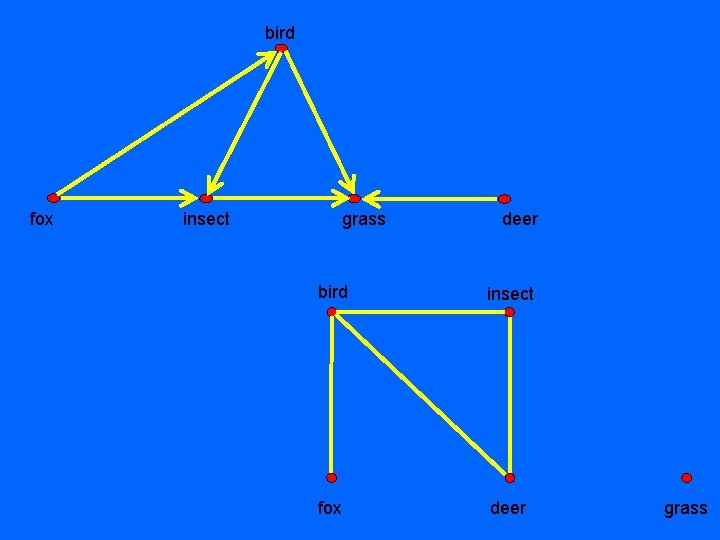bird fox insect grass deer bird insect fox deer grass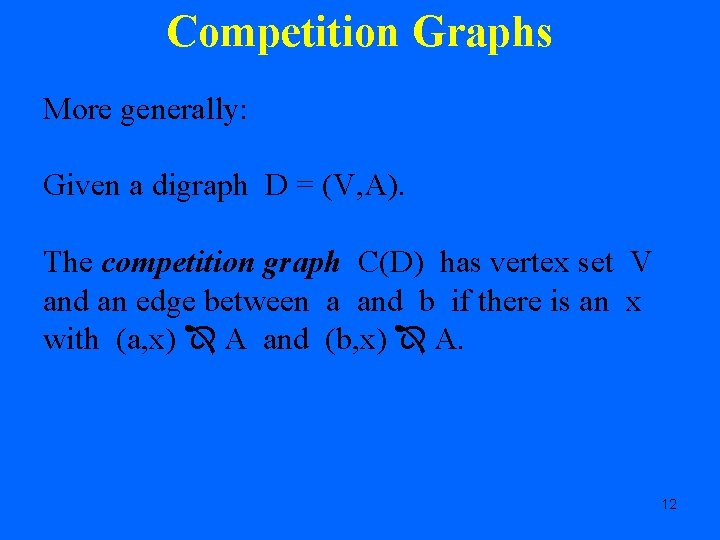Competition Graphs More generally: Given a digraph D = (V, A). The competition graph C(D) has vertex set V and an edge between a and b if there is an x with (a, x) A and (b, x) A. 12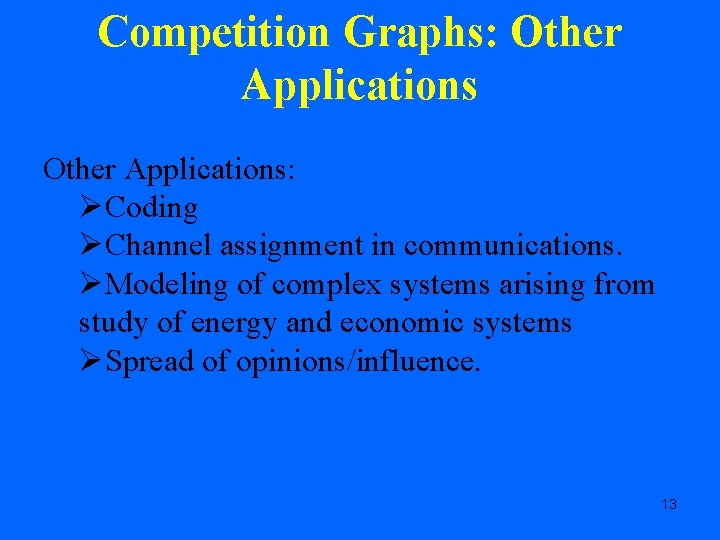Competition Graphs: Other Applications: ØCoding ØChannel assignment in communications. ØModeling of complex systems arising from study of energy and economic systems ØSpread of opinions/influence. 13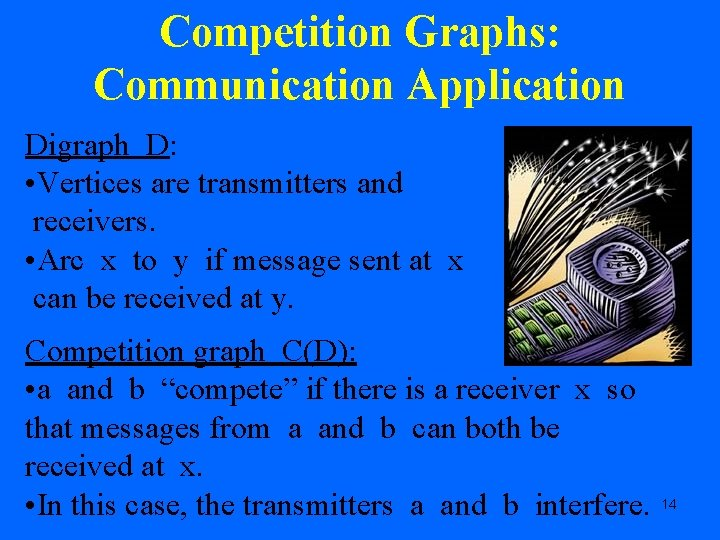Competition Graphs: Communication Application Digraph D: • Vertices are transmitters and receivers. • Arc x to y if message sent at x can be received at y. Competition graph C(D): • a and b “compete” if there is a receiver x so that messages from a and b can both be received at x. • In this case, the transmitters a and b interfere. 14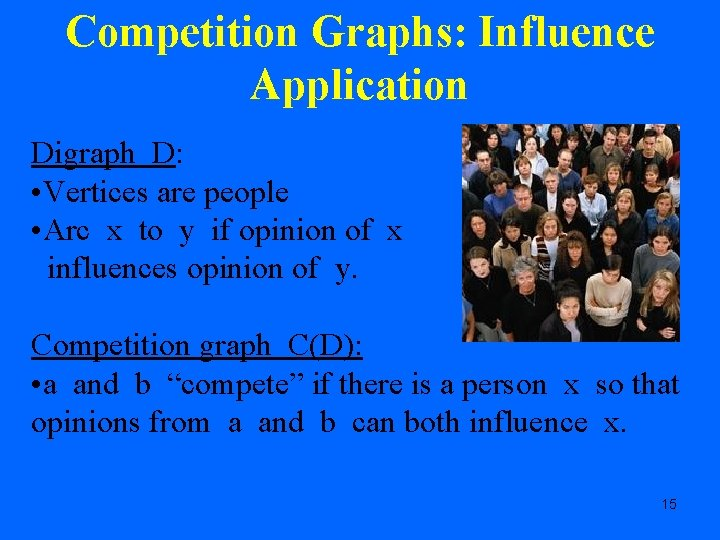Competition Graphs: Influence Application Digraph D: • Vertices are people • Arc x to y if opinion of x influences opinion of y. Competition graph C(D): • a and b “compete” if there is a person x so that opinions from a and b can both influence x. 15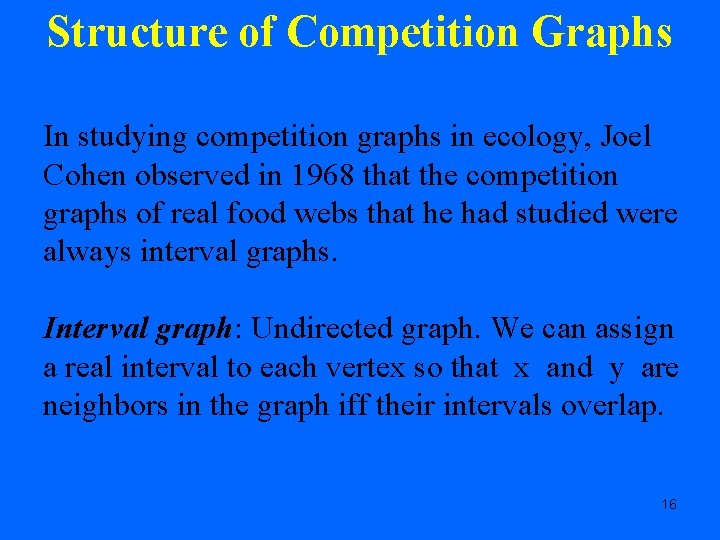Structure of Competition Graphs In studying competition graphs in ecology, Joel Cohen observed in 1968 that the competition graphs of real food webs that he had studied were always interval graphs. Interval graph: Undirected graph. We can assign a real interval to each vertex so that x and y are neighbors in the graph iff their intervals overlap. 16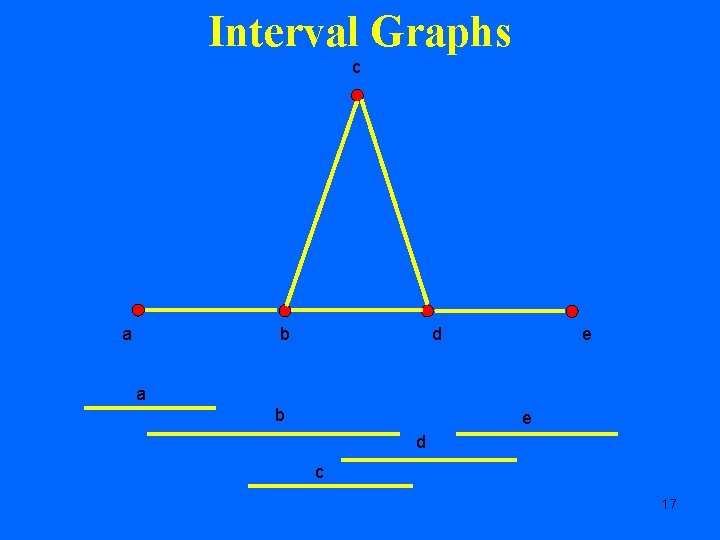Interval Graphs c a b d e a b e d c 17Structure of Competition Graphs Cohen asked if competition graphs of food webs are always interval graphs. It is simple to show that purely graphtheoretically, you can get essentially every graph as a competition graph if a food web can be some arbitrary directed graph. It turned out that there are real food webs whose competition graphs are not interval graphs, but typically not for “homogeneous” ecosystems. 18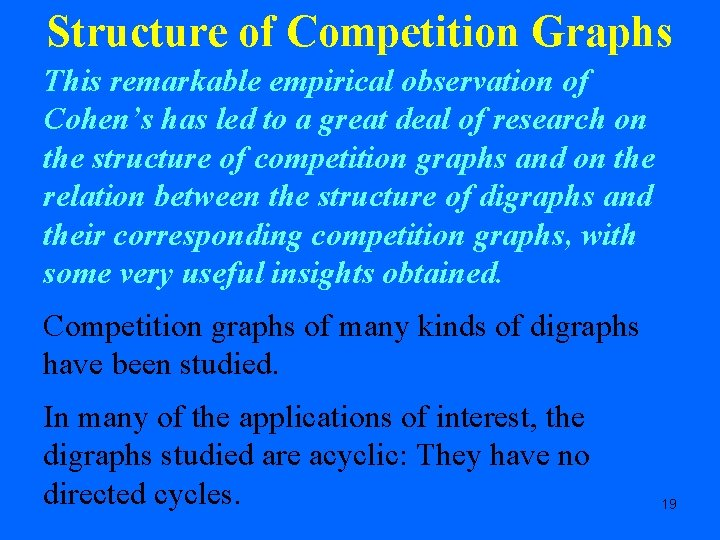Structure of Competition Graphs This remarkable empirical observation of Cohen’s has led to a great deal of research on the structure of competition graphs and on the relation between the structure of digraphs and their corresponding competition graphs, with some very useful insights obtained. Competition graphs of many kinds of digraphs have been studied. In many of the applications of interest, the digraphs studied are acyclic: They have no directed cycles. 19Structure of Competition Graphs We are interested in finding out what graphs are the competition graphs arising from semiorders. 20Competition Graphs of Semiorders Let (V, R) be a semiorder. Think of it as a digraph with an arc from x to y if x. Ry. In the communication application: Transmitters and receivers in a linear corridor and messages can only be transmitted from right to left. Because of local interference (“jamming”) a message sent at x can only be received at y if y 21 is sufficiently far to the left of x.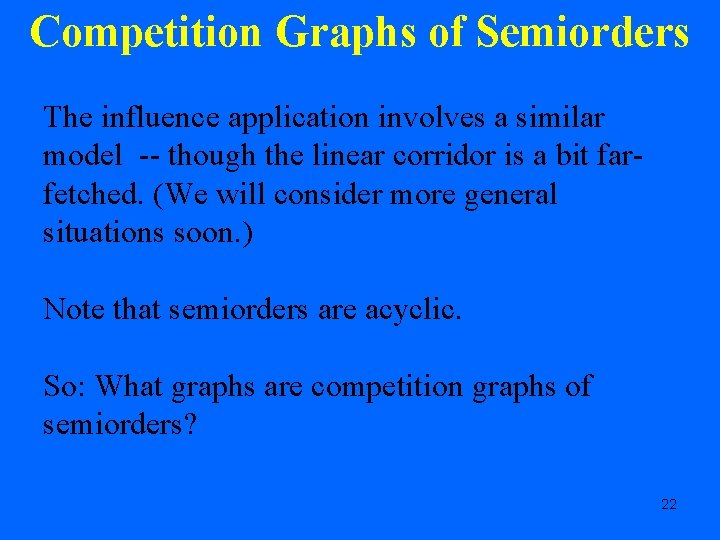Competition Graphs of Semiorders The influence application involves a similar model -- though the linear corridor is a bit farfetched. (We will consider more general situations soon. ) Note that semiorders are acyclic. So: What graphs are competition graphs of semiorders? 22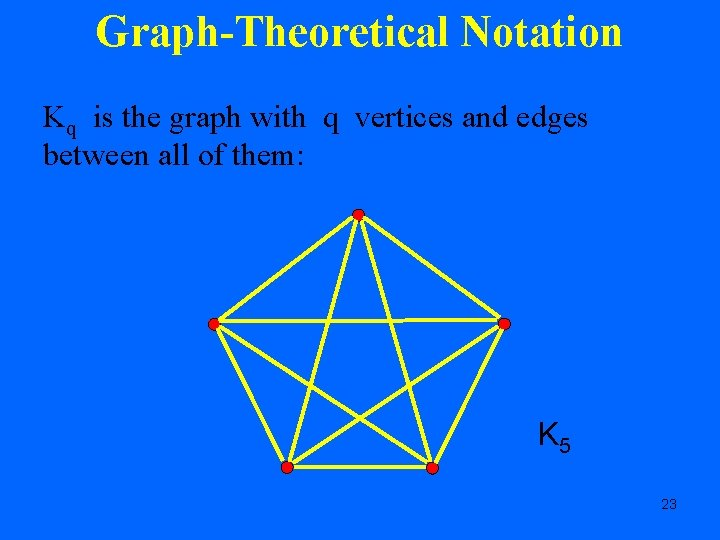Graph-Theoretical Notation Kq is the graph with q vertices and edges between all of them: K 5 23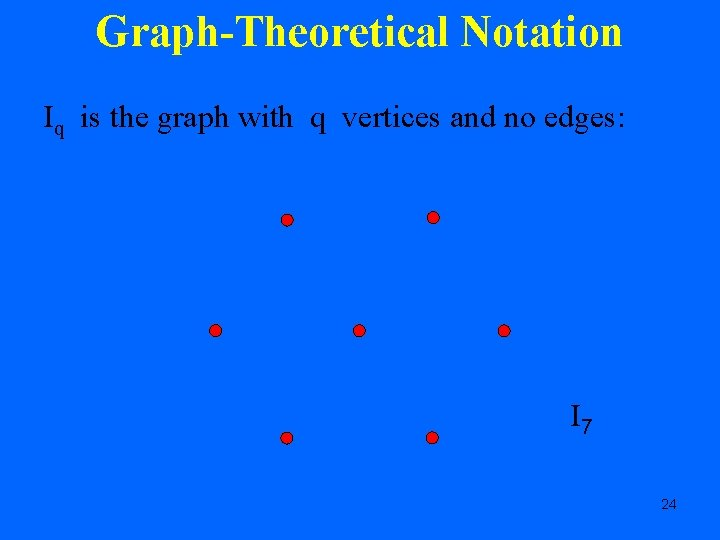Graph-Theoretical Notation Iq is the graph with q vertices and no edges: I 7 24Competition Graphs of Semiorders Theorem: A graph G is the competition graph of a semiorder iff G = Iq for q > 0 or G = Kr Iq for r >1, q > 0. Proof: straightforward. K 5 U I 7 25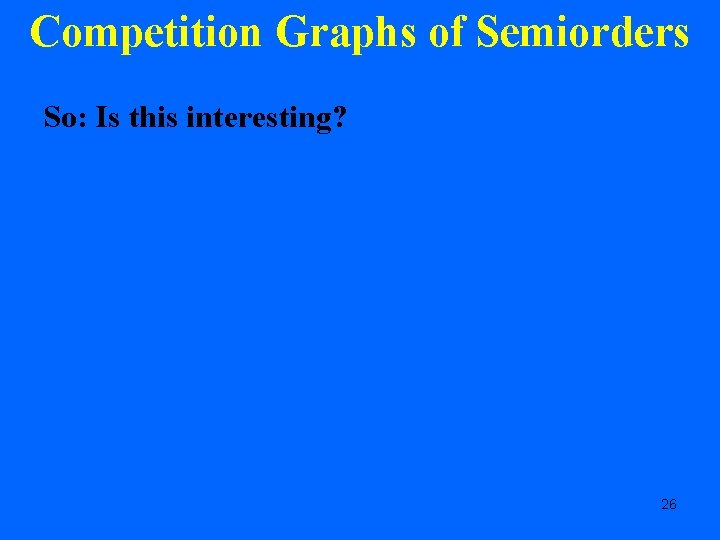Competition Graphs of Semiorders So: Is this interesting? 26Boring!Really boring!Competition Graphs of Interval Orders A similar theorem holds for interval orders. D = (V, A) is an interval order if there is an assignment of a (closed) real interval J(x) to each vertex x in V so that for all x, y V, (x, y) A J(x) is strictly to the right of J(y). Semiorders are a special case of interval orders where every interval has the same length. 29Competition Graphs of Interval Orders Theorem: A graph G is the competition graph of an interval order iff G = Iq for q > 0 or G = Kr Iq for r >1, q > 0. Corollary: A graph is the competition graph of an interval order iff it is the competition graph of a semiorder. Note that the competition graphs obtained from semiorders and interval orders are always interval graphs. We are led to generalizations. 30The Weak Order Associated with a Semiorder Given a binary relation (V, R), define a new binary relation (V, ) as follows: a b ( u)[b. Ru a. Ru & u. Ra u. Rb] It is well known that if (V, R) is a semiorder, then (V, ) is a weak order. This “associated weak order” plays an important role in the analysis of semiorders. 31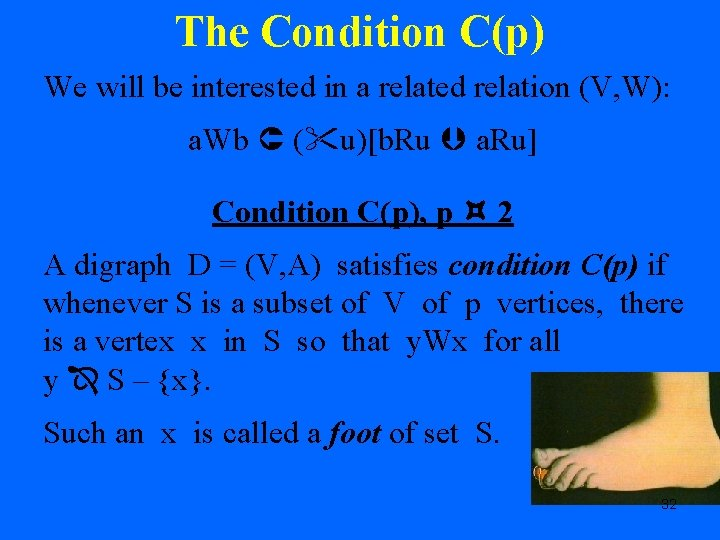The Condition C(p) We will be interested in a related relation (V, W): a. Wb ( u)[b. Ru a. Ru] Condition C(p), p 2 A digraph D = (V, A) satisfies condition C(p) if whenever S is a subset of V of p vertices, there is a vertex x in S so that y. Wx for all y S – {x}. Such an x is called a foot of set S. 32The Condition C(p) does seem to be an interesting restriction in its own right when it comes to influence. It is a strong requirement: Given any set S of p individuals in a group, there is an individual x in S so that whenever x has influence over individual u, then so do all individuals in S. 33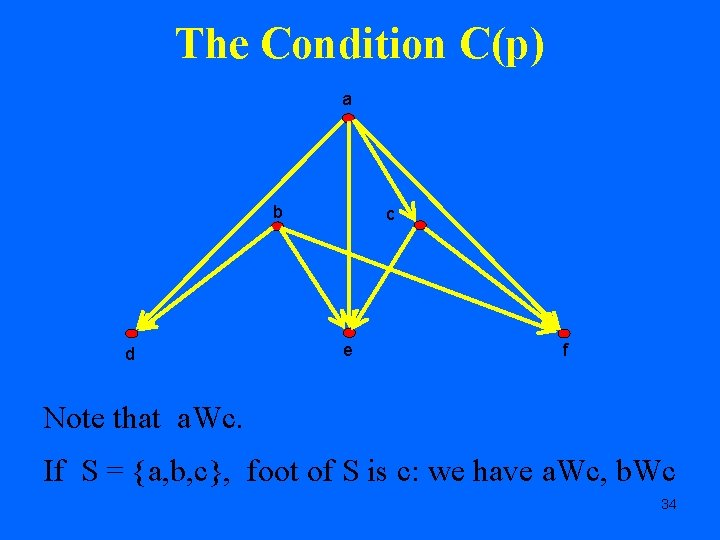The Condition C(p) a b d c e f Note that a. Wc. If S = {a, b, c}, foot of S is c: we have a. Wc, b. Wc 34The Condition C(p) Claim: A semiorder (V, R) satisfies condition C(p) for all p 2. Proof: Let f be a function satisfying: (x, y) R f(x) > f(y) + Given subset S of p elements, a foot of S is an element with lowest f-value. A similar result holds for interval orders. We shall ask: What graphs are competition graphs of acyclic digraphs that satisfy condition C(p)? 35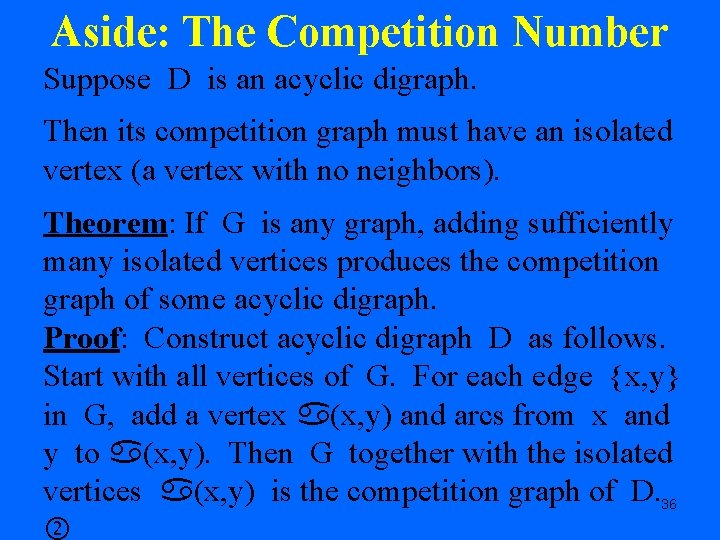Aside: The Competition Number Suppose D is an acyclic digraph. Then its competition graph must have an isolated vertex (a vertex with no neighbors). Theorem: If G is any graph, adding sufficiently many isolated vertices produces the competition graph of some acyclic digraph. Proof: Construct acyclic digraph D as follows. Start with all vertices of G. For each edge {x, y} in G, add a vertex (x, y) and arcs from x and y to (x, y). Then G together with the isolated vertices (x, y) is the competition graph of D. 36The Competition Number b a c b a d D G = C 4 α(a, b) d c a b α(b, c) α(c, d) α(a, b) α(b, c) C(D) = G U I 4 α(c, d) d α(a, d) c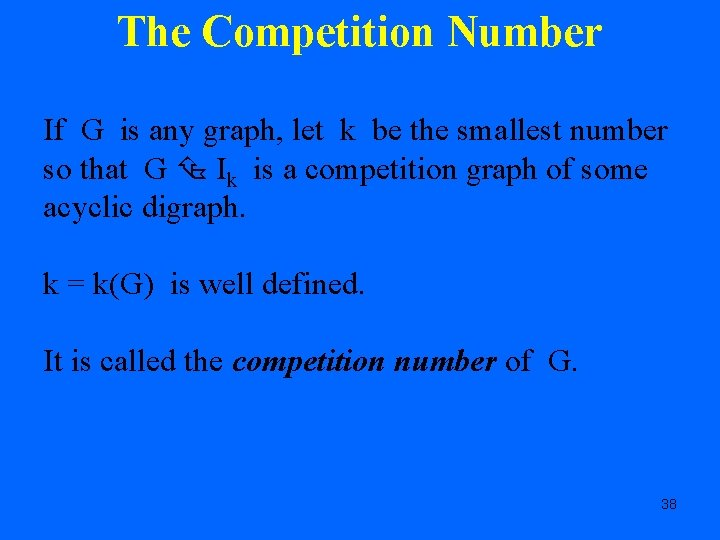The Competition Number If G is any graph, let k be the smallest number so that G Ik is a competition graph of some acyclic digraph. k = k(G) is well defined. It is called the competition number of G. 38The Competition Number Our previous construction shows that k(C 4) 4. In fact: • C 4 I 2 is a competition graph • C 4 I 1 is not • So k(C 4) = 2. 39The Competition Number Competition numbers are known for many interesting graphs and classes of graphs. However: Theorem (Opsut): It is an NP-complete problem to compute k(G). 40Competition Graphs of Digraphs Satisfying Condition C(p) Theorem: Suppose that p 2 and G is a graph. Then G is the competition graph of an acyclic digraph D satisfying condition C(p) iff G is one of the following graphs: (a). Iq for q > 0 (b). Kr Iq for r > 1, q > 0 (c). L Iq where L has fewer than p vertices, q > 0, and q k(L). 41Competition Graphs of Digraphs Satisfying Condition C(p) Note that the earlier results for semiorders and interval orders now follow since they satisfy C(2). Thus, condition (c) has to have L = I 1 and condition (c) reduces to condition (a). 42Competition Graphs of Digraphs Satisfying Condition C(p) Corollary: A graph G is the competition graph of an acyclic digraph satisfying condition C(2) iff G = Iq for q > 0 or G = Kr Iq for r >1, q > 0. Corollary: A graph G is the competition graph of an acyclic digraph satisfying condition C(3) iff G = Iq for q > 0 or G = Kr Iq for r >1, q > 0. 43Competition Graphs of Digraphs Satisfying Condition C(p) Corollary: Let G be a graph. Then G is the competition graph of an acyclic digraph satisfying condition C(4) iff one of the following holds: (a). G = Iq for q > 0 (b). G = Kr Iq for r > 1, q > 0 (c). G = P 3 Iq for q > 0, where P 3 is the path of three vertices. 44Competition Graphs of Digraphs Satisfying Condition C(p) Corollary: Let G be a graph. Then G is the competition graph of an acyclic digraph satisfying condition C(5) iff one of the following holds: (a). G = Iq for q > 0 (b). G = Kr Iq for r > 1, q > 0 Kr: r vertices, all edges (c). G = P 3 Iq for q > 0 Pr: path of r vertices (d). G = P 4 Iq for q > 0 Cr: cycle of r vertices (e). G = K 1, 3 Iq for q > 0 K 1, 3: x joined to a, b, c (f). G = K 2 Iq for q > 0 (g). G = C 4 Iq for q > 1 K 4 – e: Remove one edge (h). G = K 4 – e Iq for q > 0 45 (i). G = K 4 – P 3 Iq for q > 0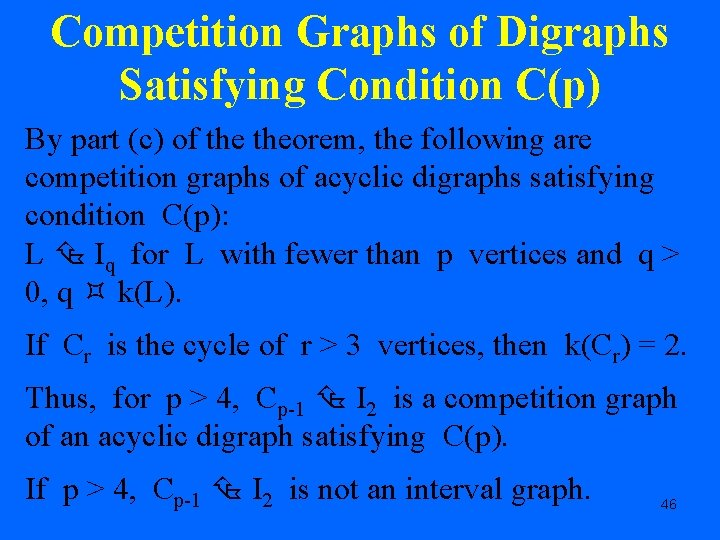Competition Graphs of Digraphs Satisfying Condition C(p) By part (c) of theorem, the following are competition graphs of acyclic digraphs satisfying condition C(p): L Iq for L with fewer than p vertices and q > 0, q k(L). If Cr is the cycle of r > 3 vertices, then k(Cr) = 2. Thus, for p > 4, Cp-1 I 2 is a competition graph of an acyclic digraph satisfying C(p). If p > 4, Cp-1 I 2 is not an interval graph. 46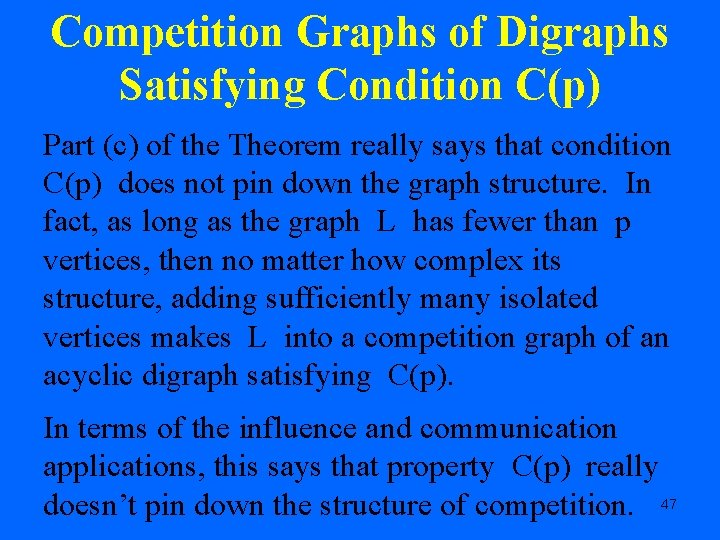Competition Graphs of Digraphs Satisfying Condition C(p) Part (c) of the Theorem really says that condition C(p) does not pin down the graph structure. In fact, as long as the graph L has fewer than p vertices, then no matter how complex its structure, adding sufficiently many isolated vertices makes L into a competition graph of an acyclic digraph satisfying C(p). In terms of the influence and communication applications, this says that property C(p) really doesn’t pin down the structure of competition. 47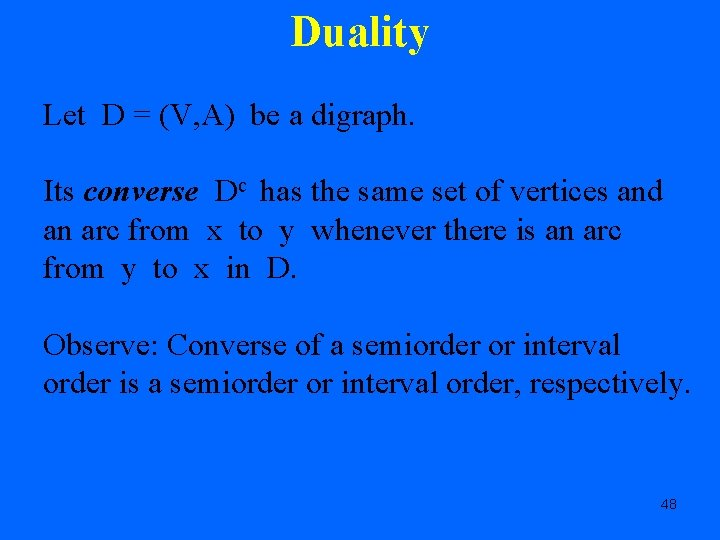Duality Let D = (V, A) be a digraph. Its converse Dc has the same set of vertices and an arc from x to y whenever there is an arc from y to x in D. Observe: Converse of a semiorder or interval order is a semiorder or interval order, respectively. 48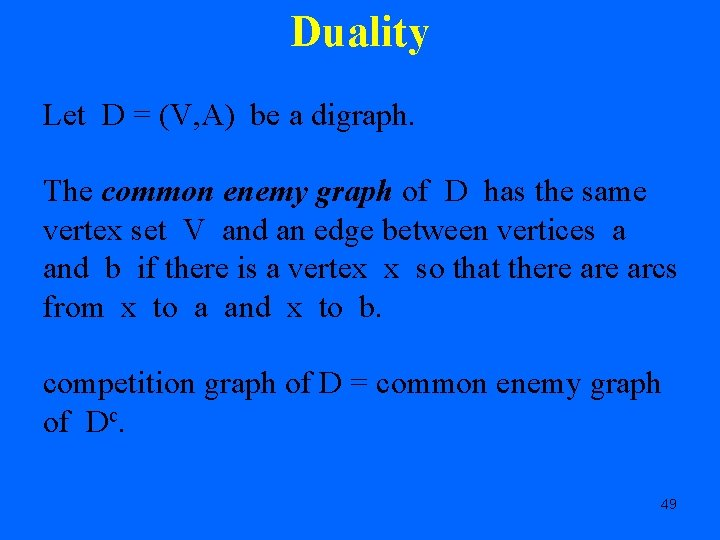Duality Let D = (V, A) be a digraph. The common enemy graph of D has the same vertex set V and an edge between vertices a and b if there is a vertex x so that there arcs from x to a and x to b. competition graph of D = common enemy graph of Dc. 49Duality Given a binary relation (V, R), we will be interested in the relation (V, W'): a. W'b ( u)[u. Ra u. Rb] Contrast the relation a. Wb ( u)[b. Ru a. Ru] Condition C'(p), p 2 A digraph D = (V, A) satisfies condition C'(p) if whenever S is a subset of V of p vertices, there is a vertex x in S so that x. W'y for all 50 y S - {x}.Duality By duality: There is an acyclic digraph D so that G is the competition graph of D and D satisfies condition C(p) iff there is an acyclic digraph D' so that G is the common enemy graph of D' and D' satisfies condition C'(p). 51Condition C*(p) A more interesting variant on condition C(p) is the following: A digraph D = (V, A) satisfies condition C*(p) if whenever S is a subset of V of p vertices, there is a vertex x in S so that x. Wy for all y S - {x}. Such an x is called a head of S. 52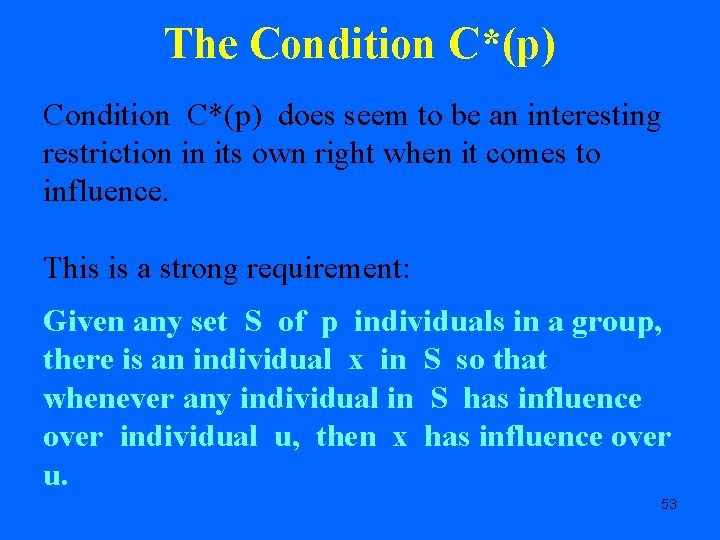The Condition C*(p) does seem to be an interesting restriction in its own right when it comes to influence. This is a strong requirement: Given any set S of p individuals in a group, there is an individual x in S so that whenever any individual in S has influence over individual u, then x has influence over u. 53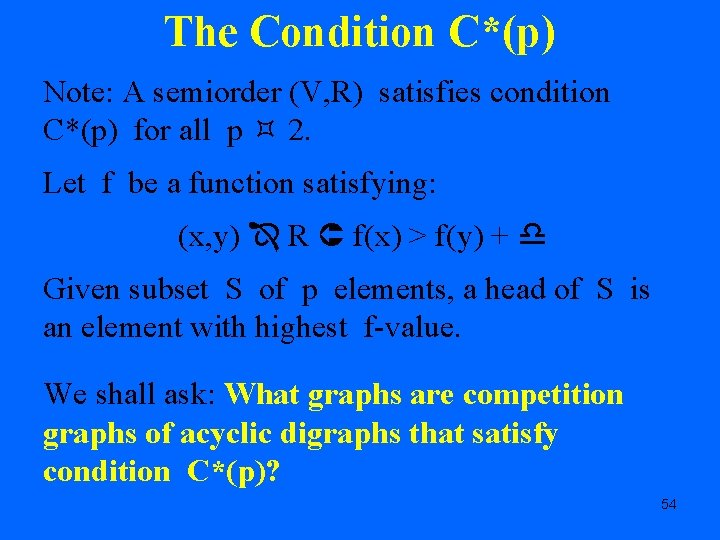The Condition C*(p) Note: A semiorder (V, R) satisfies condition C*(p) for all p 2. Let f be a function satisfying: (x, y) R f(x) > f(y) + Given subset S of p elements, a head of S is an element with highest f-value. We shall ask: What graphs are competition graphs of acyclic digraphs that satisfy condition C*(p)? 54Condition C*(p) In general, the problem of determining the graphs that are competition graphs of acyclic digraphs satisfying condition C*(p) is unsolved. We know the result for p = 2, 3, 4, or 5. 55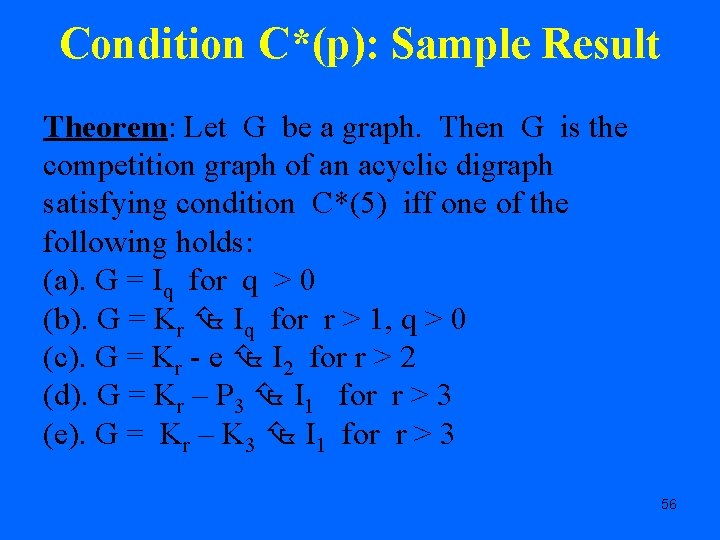Condition C*(p): Sample Result Theorem: Let G be a graph. Then G is the competition graph of an acyclic digraph satisfying condition C*(5) iff one of the following holds: (a). G = Iq for q > 0 (b). G = Kr Iq for r > 1, q > 0 (c). G = Kr - e I 2 for r > 2 (d). G = Kr – P 3 I 1 for r > 3 (e). G = Kr – K 3 I 1 for r > 3 56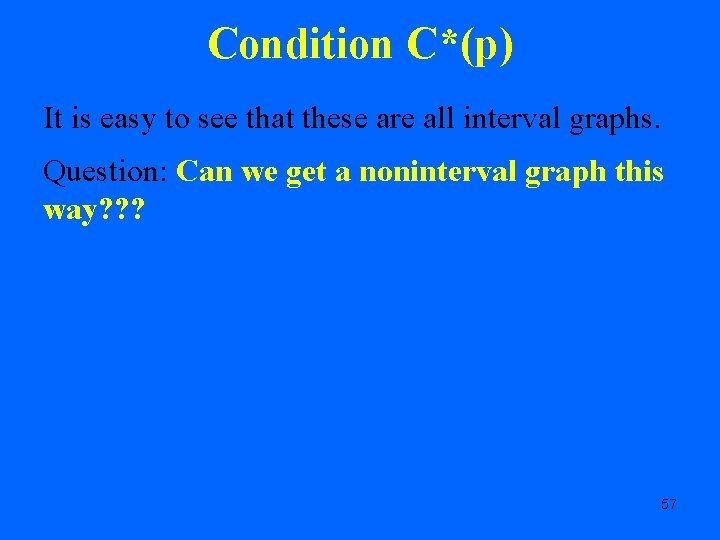Condition C*(p) It is easy to see that these are all interval graphs. Question: Can we get a noninterval graph this way? ? ? 57e d c a x b y Easy to see that this digraph is acyclic. C*(7) holds. The only set S of 7 vertices is V. Easy to see that e is a head of V. 58b a x e y d c The competition graph has a cycle from a to b to c to d to a with no other edges among {a, b, c, d}. This is impossible in an interval graph. 59Open ProblemsOpen Problems • Characterize graphs G arising as competition graphs of digraphs satisfying C(p) without requiring that D be acyclic. • Characterize graphs G arising as competition graphs of acyclic digraphs satisfying C*(p). • Determine what acyclic digraphs satisfying C(p) or C*(p) have competition graphs that are interval graphs. • Determine what acyclic digraphs satisfy conditions C(p) or C*(p). 61Closing Remark 62